13小时前
PCB Layout时，MOS管栅极串联电阻放哪儿？
2天前
LC串联谐振的意义
6天前

1星期前

1星期前# 先来思考下这么几个问题：

MOS管G极经常串联一个小电阻，说是可以抑制振荡，啥原理呢？这个电阻阻值怎么取呢？

LC串联谐振电路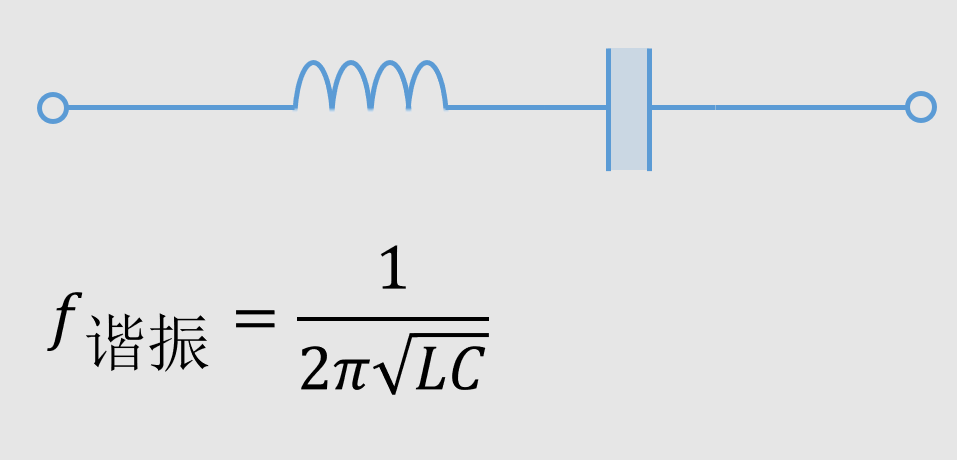LC滤波器

LC滤波器经常用，但有一个比较坑的问题就是，有时候使用LC滤波器之后，效果反而更差了，还不如不用。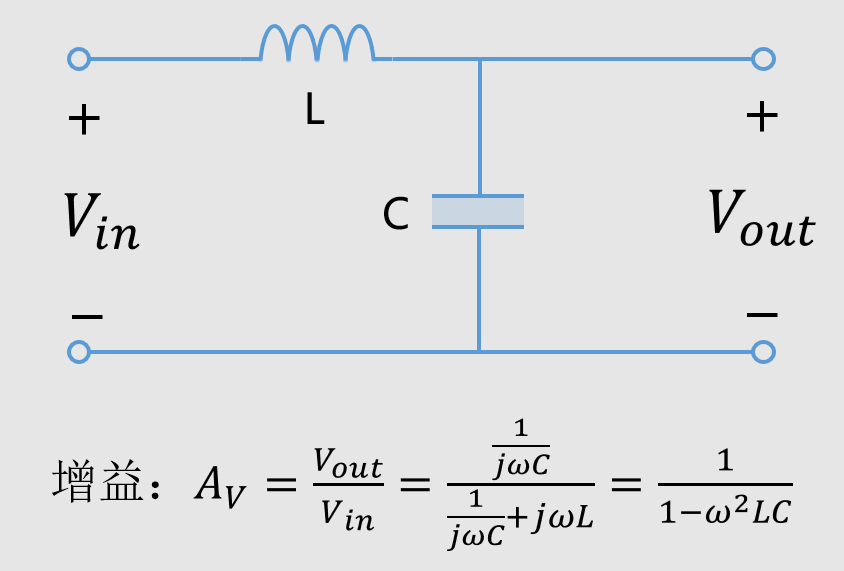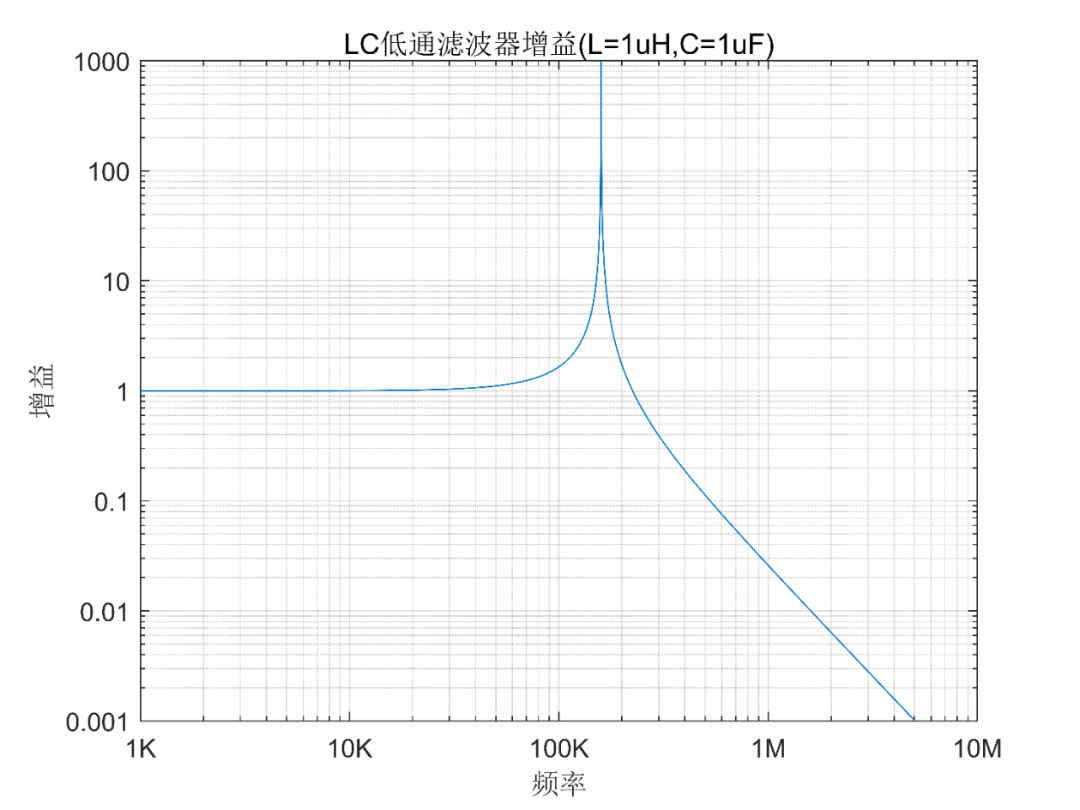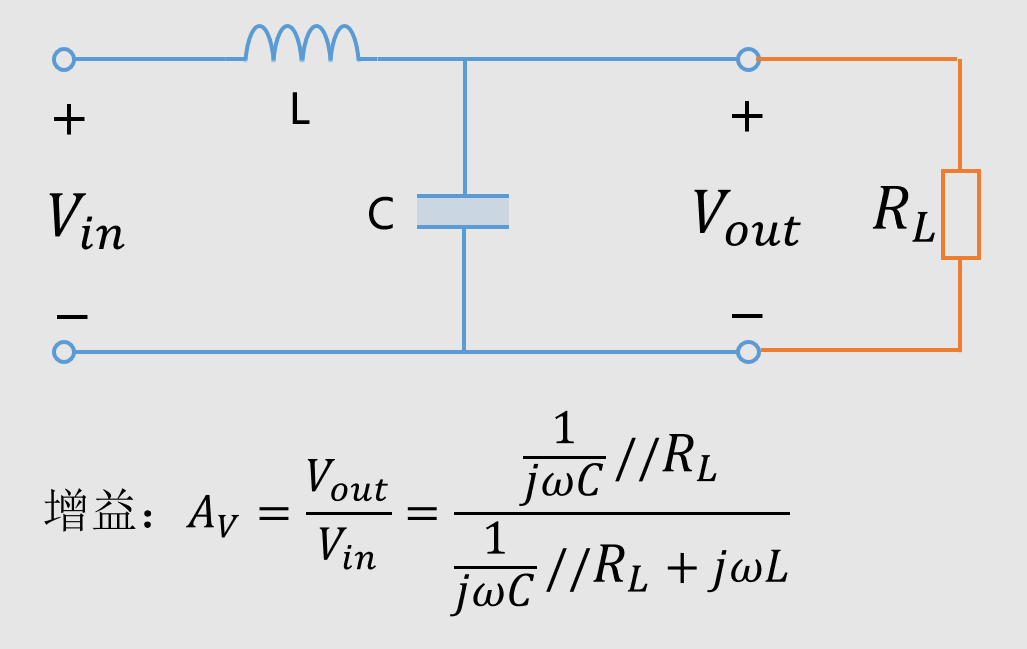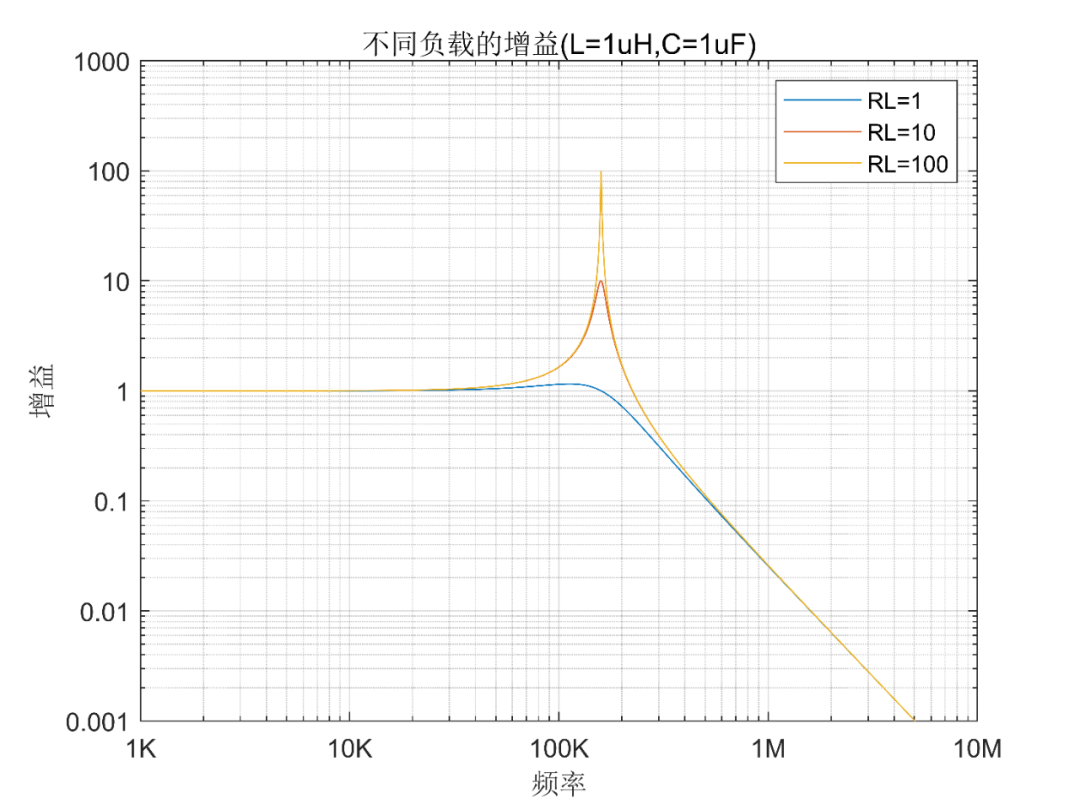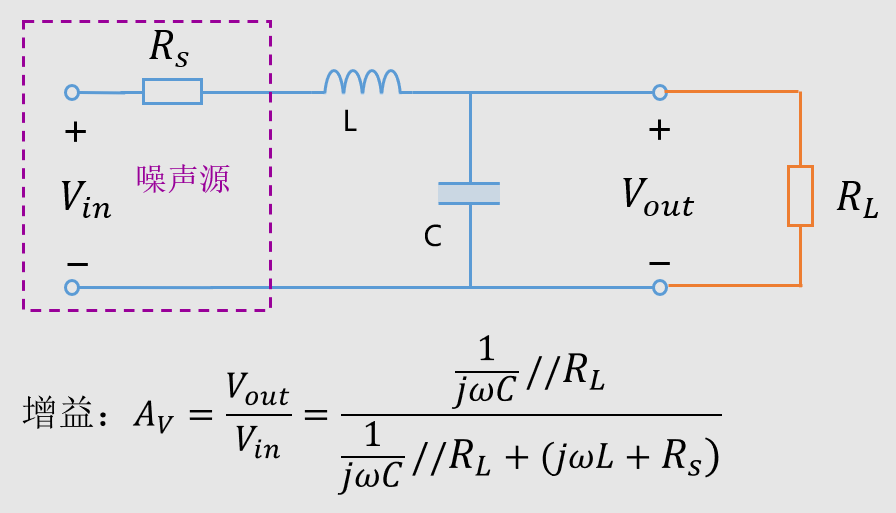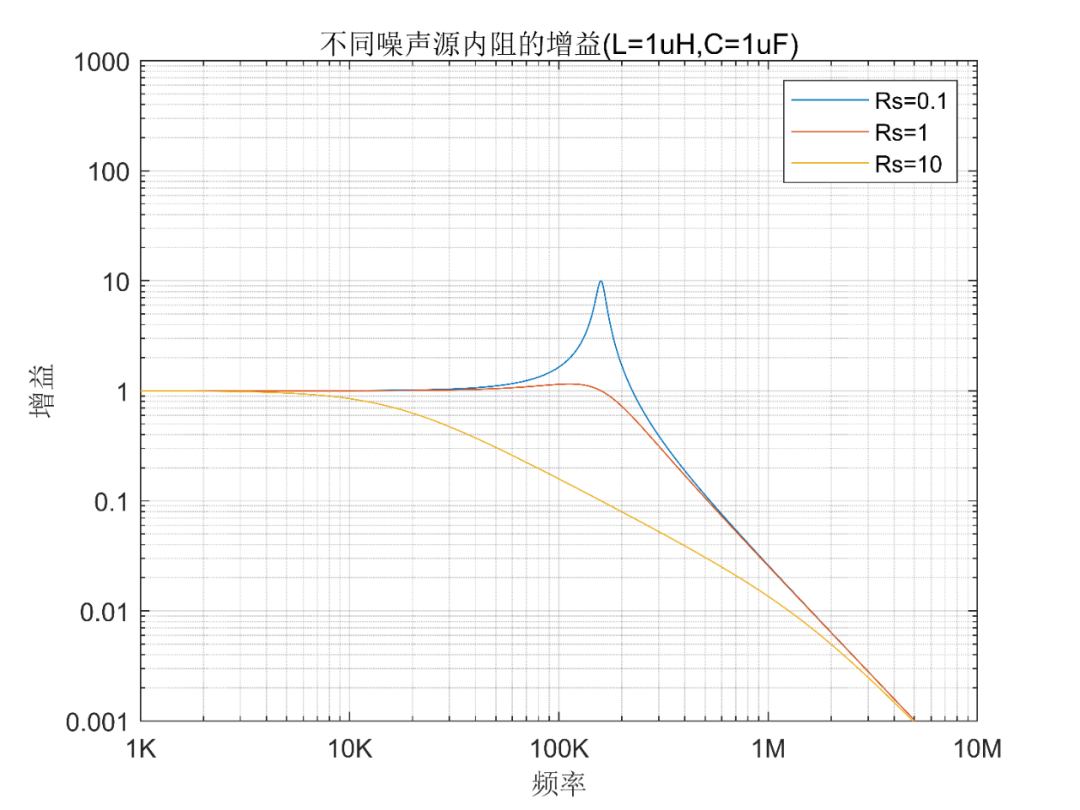L、C的值的影响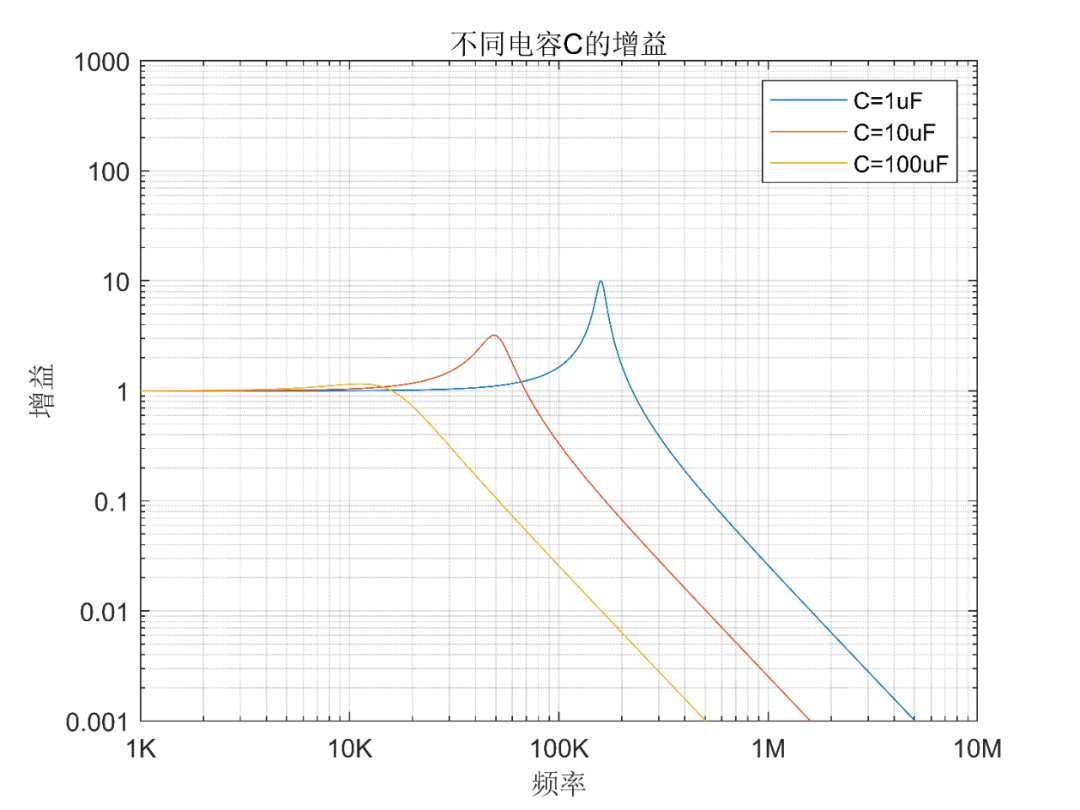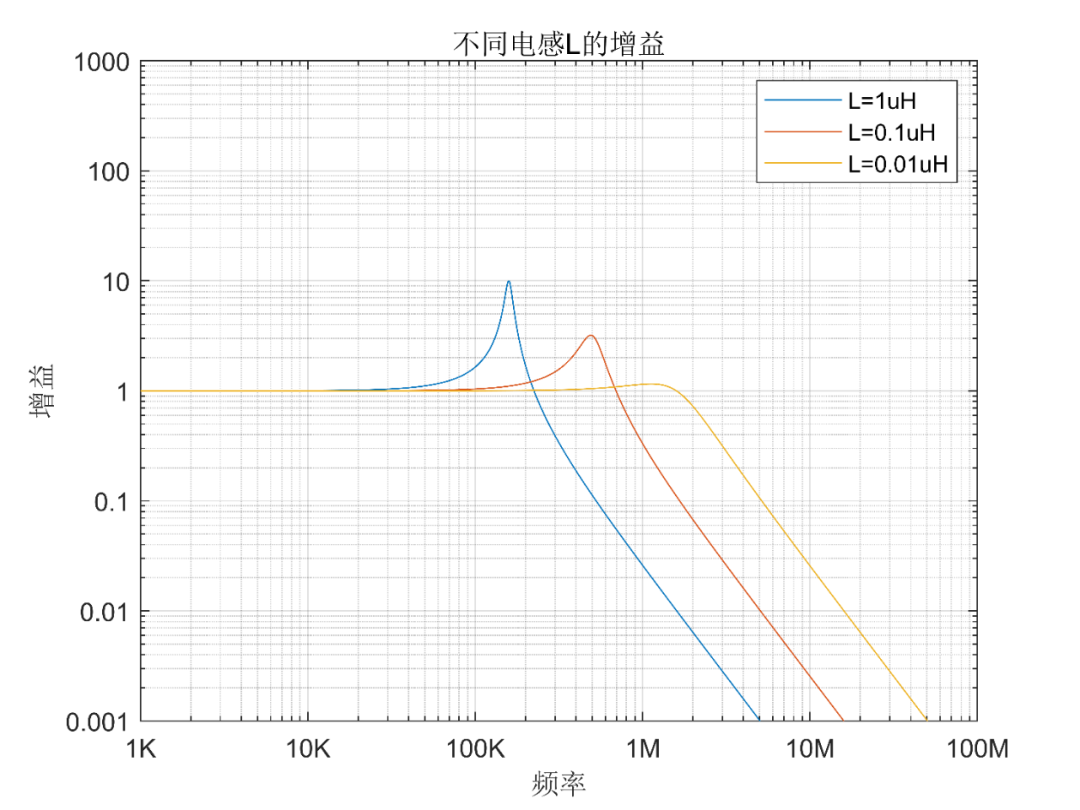MOS管G极串联电阻如何抑制谐振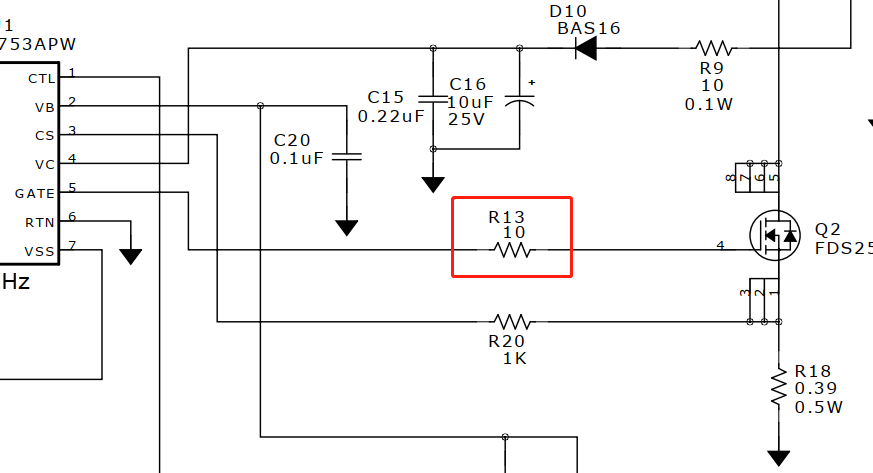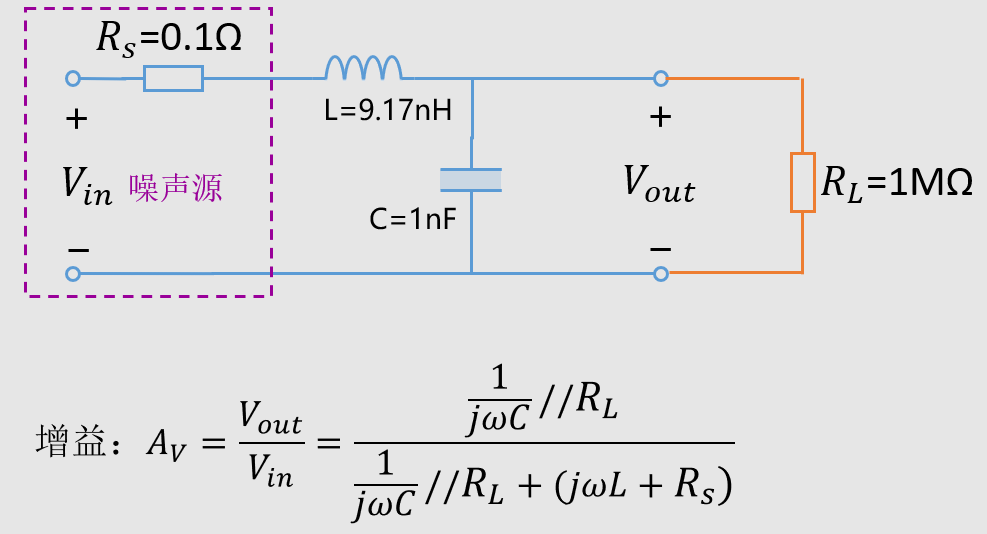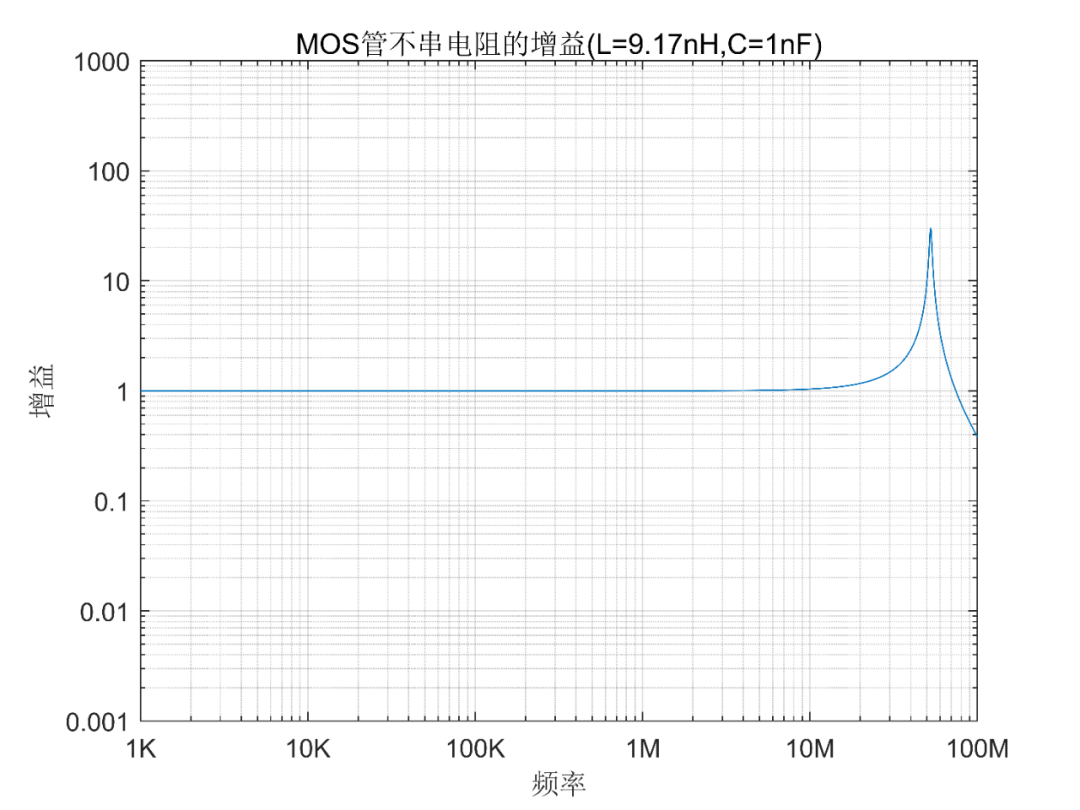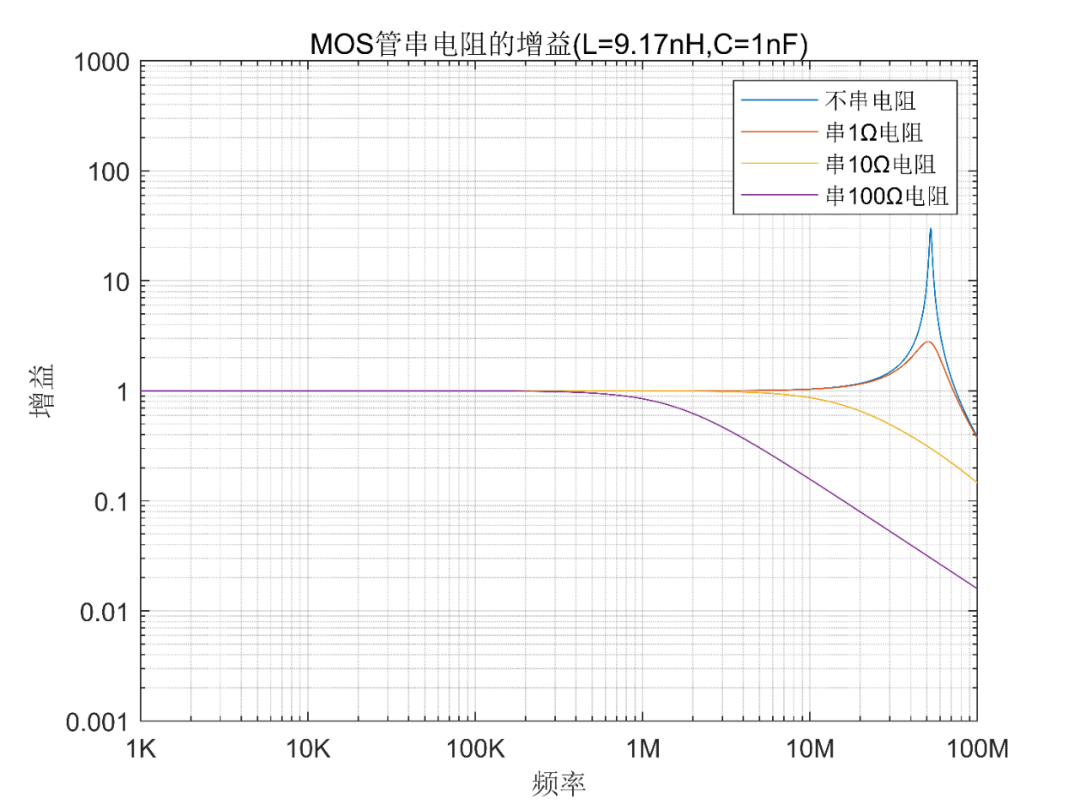Matlab源码

``````%%%%%%%%%%%%%%%%%%%%%%%%%%%%%%%%%%%%%%%
%---理想LC低通滤波器增益
%%%%%%%%%%%%%%%%%%%%%%%%%%%%%%%%%%%%%%%
f=[1000:100:100000000];   %频率：范围1Khz-10Mhz
w=(f.*pi*2);              %角频率
C=0.000001; %1uF   电容量
L=0.000001; %1uH   电感量
Zc=1./(w.*C.*j);   %电容总阻抗
Zl=w.*L.*j;        %电感总阻抗
Av= abs(Zc./(Zc+Zl));   %增益
figure;       %画图
loglog(f,Av); %画出增益曲线
grid on;      %显示网格
set(gca,'YLim',[0.001 1000]);%y轴的数据显示范围
set(gca, 'XTickLabel' ,{'1K','10K','100K','1M','10M','100M'}); %x轴频率数据
set(gca, 'YTickLabel' ,{'0.001','0.01','0.1','1','10','100','1000'});   %y轴幅度数据
xlabel('频率'), ylabel('增益');  %x，y轴名称
title(['LC低通滤波器增益(L=',num2str(L*1000000),'uH,C=',num2str(C*1000000),'uF)']);%标题

%%%%%%%%%%%%%%%%%%%%%%%%%%%%%%%%%%%%%%%
%---不同负载
%%%%%%%%%%%%%%%%%%%%%%%%%%%%%%%%%%%%%%%
f=[1000:100:100000000];   %频率：范围1Khz-10Mhz
w=(f.*pi*2);              %角频率
C=0.000001; %1uF   电容量
L=0.000001; %1uH   电感量
RL1=1;      %负载RL1=1
RL2=10;     %负载RL2=10
RL3=100;    %负载RL2=100
Zc=1./(w.*C.*j);   %电容总阻抗
Zl=w.*L.*j;        %电感总阻抗
Av1=abs(((Zc.*RL1)./(Zc+RL1))./(((Zc.*RL1)./(Zc+RL1))+Zl)); %负载1对应增益
Av2=abs(((Zc.*RL2)./(Zc+RL2))./(((Zc.*RL2)./(Zc+RL2))+Zl)); %负载2对应增益
Av3=abs(((Zc.*RL3)./(Zc+RL3))./(((Zc.*RL3)./(Zc+RL3))+Zl)); %负载3对应增益
figure;       %画图
loglog(f,Av1,f,Av2,f,Av3); %画出3种负载的增益
grid on;      %显示网格
legend(['RL=',num2str(RL1)],['RL=',num2str(RL2)],['RL=',num2str(RL3)]);%曲线说明
set(gca,'YLim',[0.001 1000]);%y轴的数据显示范围
set(gca, 'XTickLabel' ,{'1K','10K','100K','1M','10M','100M'}); %x轴频率数据
set(gca, 'YTickLabel' ,{'0.001','0.01','0.1','1','10','100','1000'});   %y轴幅度数据
xlabel('频率'), ylabel('增益');  %x，y轴名称
title(['不同负载的增益(L=',num2str(L*1000000),'uH,C=',num2str(C*1000000),'uF)']);%标题

%%%%%%%%%%%%%%%%%%%%%%%%%%%%%%%%%%%%%%%
%---不同噪声源内阻
%%%%%%%%%%%%%%%%%%%%%%%%%%%%%%%%%%%%%%%
f=[1000:100:100000000];   %频率：范围1Khz-10Mhz
w=(f.*pi*2);              %角频率
C=0.000001; %1uF   电容量
L=0.000001; %1uH   电感量
RS1=0.1;   %内阻RS1=0.1
RS2=1;     %内阻RS2=1
RS3=10;    %内阻RS2=10
RL=1000000;%负载RL=1M
Zc=1./(w.*C.*j);   %电容总阻抗
Zl=w.*L.*j;        %电感总阻抗
Av1=abs(((Zc.*RL)./(Zc+RL))./(((Zc.*RL)./(Zc+RL))+Zl+RS1)); %内阻1对应增益
Av2=abs(((Zc.*RL)./(Zc+RL))./(((Zc.*RL)./(Zc+RL))+Zl+RS2)); %内阻2对应增益
Av3=abs(((Zc.*RL)./(Zc+RL))./(((Zc.*RL)./(Zc+RL))+Zl+RS3)); %内阻3对应增益
figure;       %画图
loglog(f,Av1,f,Av2,f,Av3); %画出3种内阻的增益
grid on;      %显示网格
legend(['Rs=',num2str(RS1)],['Rs=',num2str(RS2)],['Rs=',num2str(RS3)]);%曲线说明
set(gca,'YLim',[0.001 1000]);%y轴的数据显示范围
set(gca, 'XTickLabel' ,{'1K','10K','100K','1M','10M','100M'}); %x轴频率数据
set(gca, 'YTickLabel' ,{'0.001','0.01','0.1','1','10','100','1000'});   %y轴幅度数据
xlabel('频率'), ylabel('增益');  %x，y轴名称
title(['不同噪声源内阻的增益(L=',num2str(L*1000000),'uH,C=',num2str(C*1000000),'uF)']);%标题

%%%%%%%%%%%%%%%%%%%%%%%%%%%%%%%%%%%%%%%
%---不同的电容C的值
%%%%%%%%%%%%%%%%%%%%%%%%%%%%%%%%%%%%%%%
f=[1000:100:100000000];   %频率：范围1Khz-10Mhz
w=(f.*pi*2);              %角频率
C1=0.000001; %1uF   电容量1
C2=0.00001;  %10uF   电容量2
C3=0.0001;   %100uF   电容量3
L=0.000001; %1uH   电感量
RS=0.1;   %内阻RS1=0.1
RL=1000000;%负载RL=1M
Zc1=1./(w.*C1.*j);   %电容C1总阻抗
Zc2=1./(w.*C2.*j);   %电容C2总阻抗
Zc3=1./(w.*C3.*j);   %电容C3总阻抗
Zl=w.*L.*j;          %电感总阻抗
Av1= abs(((Zc1.*RL)./(Zc1+RL))./(((Zc1.*RL)./(Zc1+RL))+Zl+RS));%电容1对应增益
Av2= abs(((Zc2.*RL)./(Zc2+RL))./(((Zc2.*RL)./(Zc2+RL))+Zl+RS));%电容2对应增益
Av3= abs(((Zc3.*RL)./(Zc3+RL))./(((Zc3.*RL)./(Zc3+RL))+Zl+RS));%电容2对应增益
figure;       %画图
loglog(f,Av1,f,Av2,f,Av3); %画出3种电容的增益
grid on;      %显示网格
legend(['C=',num2str(C1*1000000),'uF'],['C=',num2str(C2*1000000),'uF'],['C=',num2str(C3*1000000),'uF']);%曲线说明
set(gca,'YLim',[0.001 1000]);%y轴的数据显示范围
set(gca, 'XTickLabel' ,{'1K','10K','100K','1M','10M','100M'}); %x轴频率数据
set(gca, 'YTickLabel' ,{'0.001','0.01','0.1','1','10','100','1000'});   %y轴幅度数据
xlabel('频率'), ylabel('增益');  %x，y轴名称
title(['不同电容C的增益']);%标题

%%%%%%%%%%%%%%%%%%%%%%%%%%%%%%%%%%%%%%%
%---不同的电感L的值
%%%%%%%%%%%%%%%%%%%%%%%%%%%%%%%%%%%%%%%
f=[1000:100:100000000];   %频率：范围1Khz-10Mhz
w=(f.*pi*2);              %角频率
C=0.000001; %1uF   电容量
L1=0.000001; %1uH   电感量
L2=0.0000001; %0.1uH   电感量
L3=0.00000001; %0.01uH   电感量
RS=0.1;   %内阻RS1=0.1
RL=1000000;%负载RL=1M
Zc=1./(w.*C.*j);   %电容C总阻抗
Zl1=w.*L1.*j;          %电感L1总阻抗
Zl2=w.*L2.*j;          %电感L2总阻抗
Zl3=w.*L3.*j;          %电感L3总阻抗
Av1= abs(((Zc.*RL)./(Zc+RL))./(((Zc.*RL)./(Zc+RL))+Zl1+RS));%电感1对应增益
Av2= abs(((Zc.*RL)./(Zc+RL))./(((Zc.*RL)./(Zc+RL))+Zl2+RS));%电感2对应增益
Av3= abs(((Zc.*RL)./(Zc+RL))./(((Zc.*RL)./(Zc+RL))+Zl3+RS));%电感3对应增益
figure;       %画图
loglog(f,Av1,f,Av2,f,Av3); %画出3种电感的增益
grid on;      %显示网格
legend(['L=',num2str(L1*1000000),'uH'],['L=',num2str(L2*1000000),'uH'],['L=',num2str(L3*1000000),'uH']);%曲线说明
set(gca,'YLim',[0.001 1000]);%y轴的数据显示范围
set(gca, 'XTickLabel' ,{'1K','10K','100K','1M','10M','100M'}); %x轴频率数据
set(gca, 'YTickLabel' ,{'0.001','0.01','0.1','1','10','100','1000'});   %x轴幅度数据
xlabel('频率'), ylabel('增益');  %x，y轴名称
title(['不同电感L的增益']);%标题

%%%%%%%%%%%%%%%%%%%%%%%%%%%%%%%%%%%%%%%
%---MOS不串电阻
%%%%%%%%%%%%%%%%%%%%%%%%%%%%%%%%%%%%%%%
f=[1000:100:100000000];   %频率：范围1Khz-10Mhz
w=(f.*pi*2);              %角频率
C=0.000000001; %1nF   电容量
L=0.00000000917; %1uH   电感量
RS=0.1;   %内阻RS1=0.1
RL=1000000;%负载RL=1M
Zc=1./(w.*C.*j);   %电容总阻抗
Zl=w.*L.*j;        %电感总阻抗
Av=abs(((Zc.*RL)./(Zc+RL))./(((Zc.*RL)./(Zc+RL))+Zl+RS)); %MOS管不串增益
figure;       %画图
loglog(f,Av); %画出增益曲线
grid on;      %显示网格
set(gca,'YLim',[0.001 1000]);%y轴的数据显示范围
set(gca, 'XTickLabel' ,{'1K','10K','100K','1M','10M','100M'}); %x轴频率数据
set(gca, 'YTickLabel' ,{'0.001','0.01','0.1','1','10','100','1000'});   %x轴幅度数据
xlabel('频率'), ylabel('增益');  %x，y轴名称
title(['MOS管不串电阻的增益(L=',num2str(L*1000000000),'nH,C=',num2str(C*1000000000),'nF)']);%标题

%%%%%%%%%%%%%%%%%%%%%%%%%%%%%%%%%%%%%%%
%---MOS串电阻
%%%%%%%%%%%%%%%%%%%%%%%%%%%%%%%%%%%%%%%
f=[1000:100:100000000];   %频率：范围1Khz-10Mhz
w=(f.*pi*2);              %角频率
C=0.000000001; %1nF   电容量
L=0.00000000917; %1uH   电感量
RS1=0.1;   %内阻RS1=0.1
RS2=1.1;   %内阻RS1=1.1
RS3=10.1;   %内阻RS1=10.1
RS4=100.1;   %内阻RS1=100.1
RL=1000000;%负载RL=1M
Zc=1./(w.*C.*j);   %电容总阻抗
Zl=w.*L.*j;        %电感总阻抗
Av1=abs(((Zc.*RL)./(Zc+RL))./(((Zc.*RL)./(Zc+RL))+Zl+RS1)); %MOS管不串增益
Av2=abs(((Zc.*RL)./(Zc+RL))./(((Zc.*RL)./(Zc+RL))+Zl+RS2)); %MOS管串1Ω电阻增益
Av3=abs(((Zc.*RL)./(Zc+RL))./(((Zc.*RL)./(Zc+RL))+Zl+RS3)); %MOS管串1Ω电阻增益
Av4=abs(((Zc.*RL)./(Zc+RL))./(((Zc.*RL)./(Zc+RL))+Zl+RS4)); %MOS管串1Ω电阻增益
figure;       %画图
loglog(f,Av1,f,Av2,f,Av3,f,Av4); %画出增益曲线
grid on;      %显示网格
legend(['不串电阻'],['串1Ω电阻'],['串10Ω电阻'],['串100Ω电阻']);%曲线说明
set(gca,'YLim',[0.001 1000]);%y轴的数据显示范围
set(gca, 'XTickLabel' ,{'1K','10K','100K','1M','10M','100M'}); %x轴频率数据
set(gca, 'YTickLabel' ,{'0.001','0.01','0.1','1','10','100','1000'});   %x轴幅度数据
xlabel('频率'), ylabel('增益');  %x，y轴名称
title(['MOS管串电阻的增益(L=',num2str(L*1000000000),'nH,C=',num2str(C*1000000000),'nF)']);%标题
``````

LC串联电路非常简单，然而实际电路应用起来却不简单，从而会引起各种各样的现象，如果不深入分析的话，确实会有点无从下手。下面写几个小结论：

1、LC串联谐振的增益，与信源内阻，负载阻抗，电感，电容的大小都有很大的关系，四个变量造成的情景组合非常多，表现也就有很不一样。总的来说信源内阻越小，负载阻抗越大，电感越大，电容越小，越容易出现尖峰

2、LC滤波器恶化要满足几个条件：源内阻要小，负载阻抗要大，噪声频率正好处于谐振频率附近，电容容量太小，电感感量太大。

0/200